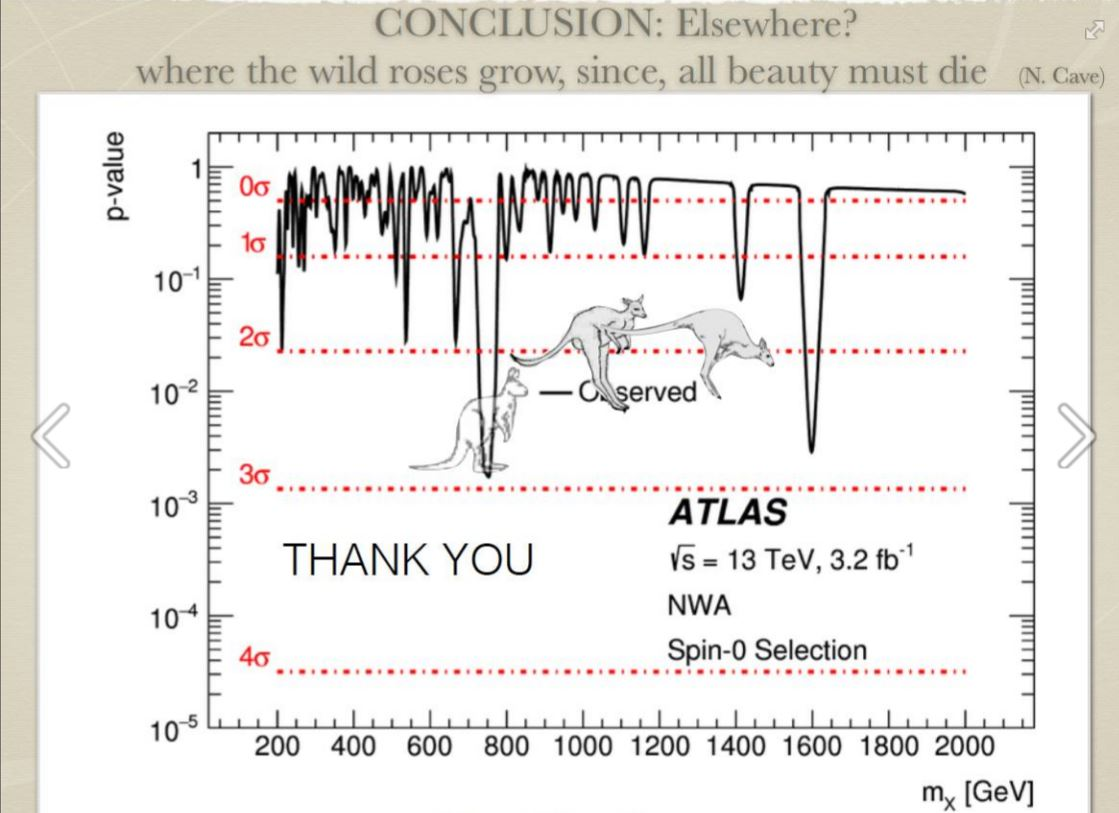## Tuesday, July 05, 2016

### Gerardus 't Hooft and string theory

Gerardus 't Hooft is celebrating his 70th birthday today. Congratulations!(C) LM

He got his 1999 Nobel prize for the proof of the renormalizability of the electroweak theory, along with Martinus Veltman, his adviser, whose main contribution was to assign his powerful student with a good and ambitious homework exercise. Because of his magical technical skills, 't Hooft used to be nicknamed The Ayatollah – just because most of his colleagues didn't realize that The Shah was actually much better than the Persian mullah-in-chief.

Even though 't Hooft has taught a string theory course at his university, I think people would agree that he's not a string theorist. However, a modern look at his contributions is a good example of the high degree of organization and clarification that string theory has introduced to theoretical physics.

First, the proof of the renormalizability of gauge theories doesn't have an immediate relationship with string theory because gauge theories "are not" string theory. Except that in some superselection sectors, they are. The AdS/CFT correspondence implies that string theory on anti de Sitter-based backgrounds may be reduced to the low-energy limit of the D-brane dynamics which is simply a gauge theory. Because string theory is nice and finite, the relevant limit should have the same property. And it has.

't Hooft was also the first man, ahead of Leonardus Susskind, who has revealed the heuristic arguments in favor of the holographic principle, a more vague but more general idea than one demonstrated in the AdS/CFT correspondence.

The 't Hooft interaction coupling many fermions whose zero modes appear as solutions on top of an instanton has some interpretations or generalizations in terms of D-instantons and other objects in string theory.

He is also rumored to be the first guy who (in 1971) calculated the beta-function of QCD, including the $$-11/3$$ factor, before Gross, Wilczek, and Politzer did. But he didn't publish it. The factor of $$11=22/2$$ may be interpreted as the argument of $$SO(22)$$, the isometry of the sphere in $$AdS^5 \times S^{21}$$, a background for the $$D=26$$ string theory that is closer to a solution than it would be in other dimensions. The appearance of the number $$11$$ and the $$D=26$$ critical dimension of bosonic string theory aren't independent facts.

However, 't Hooft's most cited paper – also and perhaps especially by string theorists – is A planar diagram theory for strong interactions, with over 4,000 citations. The 't Hooft limit is mentioned in thousands of string theory papers, of course.

If you consider Feynman diagrams for gauge theories with adjoint fields, they look like this:The propagators are lines that are conveniently refined as "double lines" because these fields and particles carry two fundamental $$SU(N)$$ indices, for example $$j,k$$. With a big density of lines, the Feynman diagrams may approximate surfaces. Every time you cut an area by a non-twisted propagator (a double line) – imagine e.g. that you cut the brown area in the middle by an extra horizontal line – you add two cubic vertices i.e. a factor of $$g^2$$ but you also increase the number of faces by one. So the parametric dependence gains an extra $$\lambda=g^2 N$$, the 't Hooft coupling, but no additional factor of $$1/N$$. If you added "handles" to the area, the terms would be suppressed by an additional factor of $$1/N$$, much like if you twisted the horizontal line we just mentioned so that the two boundaries of the two successor states would be joined into one.

So in the large $$N$$ limit with a large but fixed $$\lambda = g^2 N$$, the gauge theory has to look like a world sheet of string theory. And the AdS/CFT correspondence in the "gauge-gravity duality" version makes this equivalence rigorous.

I want to mention one point. You could object that the "exact theory" for a finite $$g,N$$ has to have "either" the continuous world sheets as imagined by string theory; "or" the triangulated world sheets as obtained from the gauge theory Feynman diagrams. Which of them is right? Surely just one may be right, you might say.

The funny thing is that there is no contradiction because the stringy world sheet is described by a theory of quantum gravity (in 2 spacetime i.e. world sheet dimensions) itself. So the "bulk" doesn't really exist. I believe that there's a way to rigorously prove – and I have partly written down the proof – that the perturbative expansion of the $$AdS_5\times S^5$$ type IIB string theory coincides with the perturbative expansion of the $$\NNN=4$$ Yang-Mills theory.Off-topic, cernette: ATLAS' Eilam Gross' slide at SUSY 2016. The kangaroo may be a hint about what happened with the cernette. ;-) Song.

The gauge theory propagators are matched to the points of the stringy world sheet where it touches the boundary of a hyperbolic plane (Poincaré disk), a Euclideanized $$AdS_2$$. So all the faces of the Feynman diagram drawn on the string world sheet are actually examples of quantum gravity in $$EAdS_2$$. By holography, the dynamics of this gravitational theory may be reduced to the conformal theory in a dimension smaller by one, i.e. $$ECFT_1$$, and that's the dynamics producing the propagators (and hopefully also the Feynman vertices where 3+ faces meet).

This removal of one world sheet dimension through the "holography on the world sheet" may be viewed as a general heuristic explanation why some sectors of string theory are equivalent to a quantum field theory.